# Angular Momentum - Rotation About Fixed Axis

If we consider an extended object as a system of small particles constituting its body, the rate of change of angular momentum with respect to time of the system of particles at a fixed point is equal to the total external torque acting on the system about that point. Can we calculate the net rate of change of angular momentum of a rotating object about a fixed axis? In this section, we will learn about the angular momentum of a particle undergoing rotational motion about a fixed axis.

Angular momentum for an object rotating about a fixed axisConsider an object rotating about a fixed axis, as shown in the figure. Let us consider a particle P in the body that rotates about the axis as shown above. The expression of total angular momentum for this system can be given by,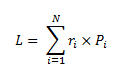Where P is the momentum of the particle (which is equal to mv) and r is the distance of the particle from the axis of rotation.

The contribution of an individual particle to the total angular momentum can be given as,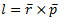Here the vector r is equal to OP, and we can write OP = OC + CP.

So we can write,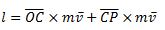Here,where rp is the perpendicular distance of the point P from the axis of rotation.

As we can see the tangential velocity v at the point p is perpendicular to the vector rp. Using the right-hand thumb rule, we can say that the direction of product CP×v is parallel to the axis of rotation.

Similarly, we can see that the product of the vectors OC×V is perpendicular to the axis of rotation.

So, we can write,Where Iz is the component of the angular momentum parallel to the fixed axis of rotation, which is along the z-axis.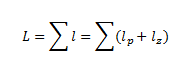The perpendicular component of momentum Lp can be given as,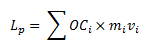And the parallel component of the momentum can be given as,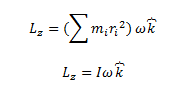The object under consideration is generally symmetric about the axis of rotation; hence at a particular perpendicular distance rp, each and every particle possessing a velocity vi has a corresponding particle possessing velocity –vi located diametrically opposite on the circle. Hence, the total angular momentum due to these particles sums up to zero, as they cancel each other.

For such symmetrical objects, the total momentum of the object is given by,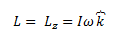Where, I is the moment of inertia of the body, ω is the angular velocity of the body and gives the direction of the total angular momentum.